# Arithmetic and Logic Operators

10.07.2017

Episode #6 of the course Coding сoncepts with Scratch by Alan Cohen

Scratch provides you the tools to perform a variety of operations. This lesson introduces you to arithmetic and logical operations.

Arithmetic Operations

Arithmetic operations allow you to code programs that add, subtract, multiply, and divide.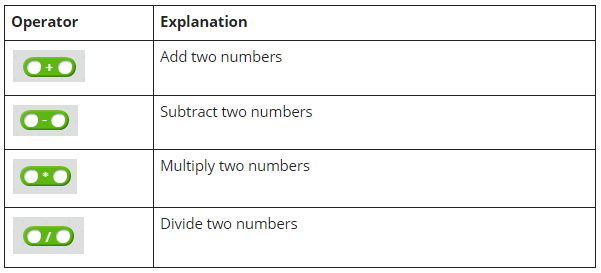You can use both numbers and variables. Here are a few examples.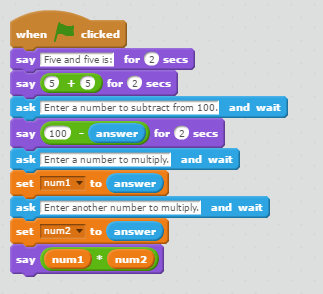The first example adds the numbers that the operator contains.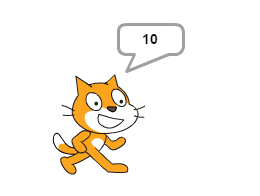The second example asks the user to enter a number to subtract from 100. The subtraction operation performs this request using a number and a variable.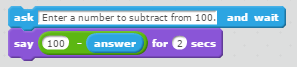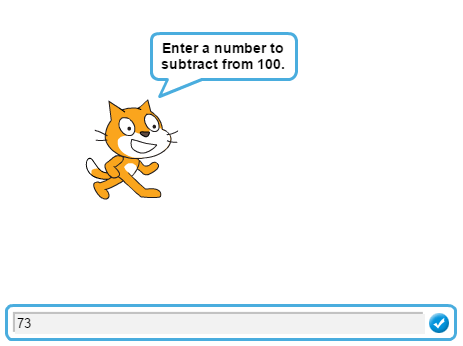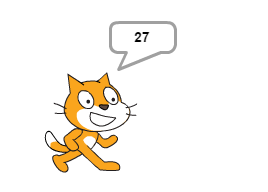The last example asks the user to enter two numbers to multiply. Both numbers are stored in separate variables. The multiplication operation uses these variables to perform the calculation.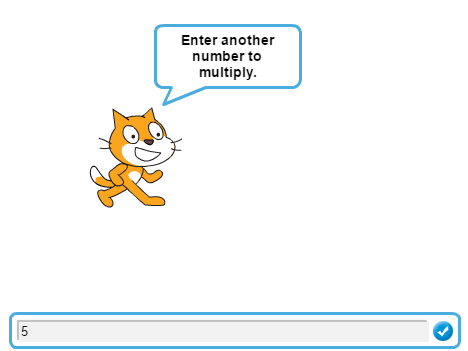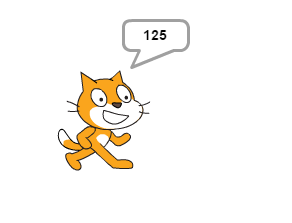Scratch also provides operators to compare numbers.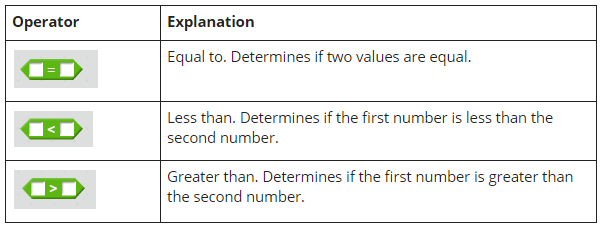The following program is a number guessing game.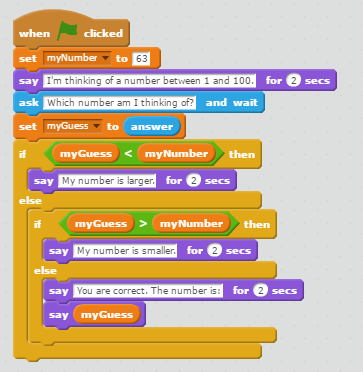The program tells the user that it is thinking of a number between 1 and 100 and asks the user to enter a guess. The program checks to see if the guess is less than its number. If it is, a message displays that the number is larger than your guess.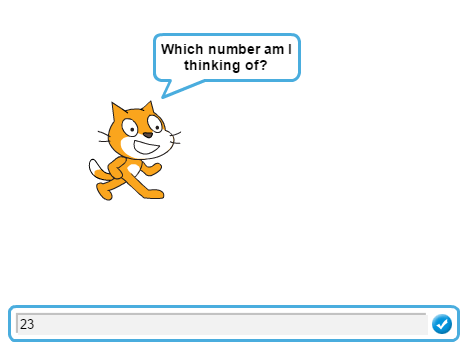If the user’s guess is not less than the number, the program checks to see if the guess is larger than its number. If it is, a message displays that the number is smaller than your guess.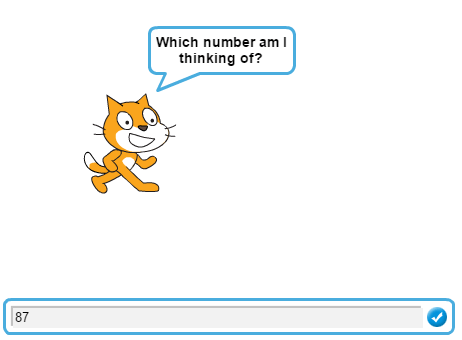If your guess is not less than and is not greater than the number, both numbers must be equal. The program tells you that you are correct and displays its number.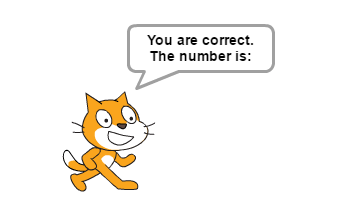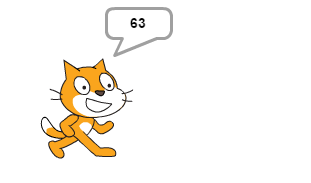Logical Operators

Logical operators test whether conditions are true or false. There are three logical operators, AND, OR, and NOT.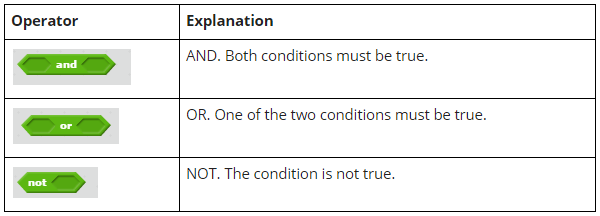OR Example

Let’s modify our number guessing program to test if the number is both less than or greater than the program’s number using the OR operator. Change the logic in the IF-Then-Else statement to check to see if the guess is either smaller or larger than the program’s number.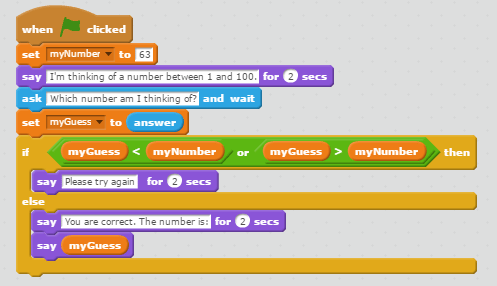If the guess is less than or greater than the number, the user did not guess the correct number.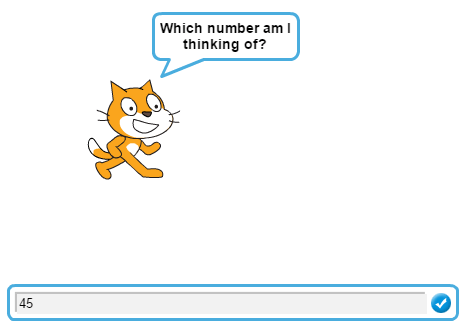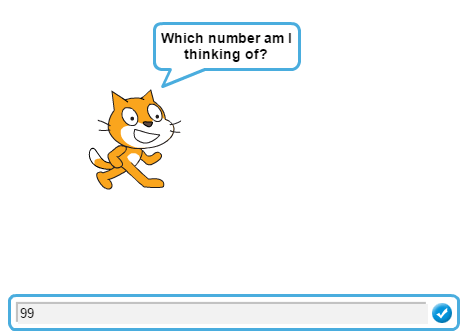Otherwise, they guessed the correct number.Using the AND Operator and the NOT Operator

This example modifies the number guessing program to use both the AND operator and the NOT operator.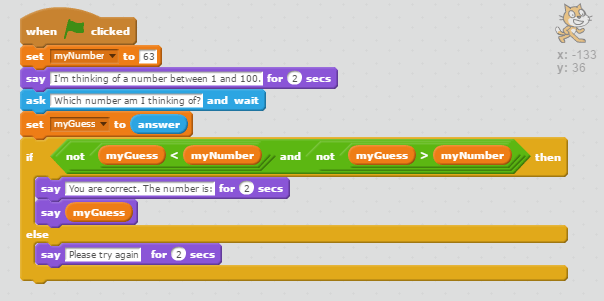When you compare two numbers, if the number is neither less than nor greater than the other number, the only choice left is that it’s equal to the other number.If the guess is less than or greater than the number, the user did not guess the correct number.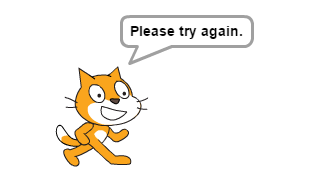So, now you know how to use arithmetic and logic operators. In the next lesson, you will learn about text operators.

Recommended book

Scratch Programming in Easy Steps by Sean McManus

Share with friends